# Algebra I EOC STAAR Review Activities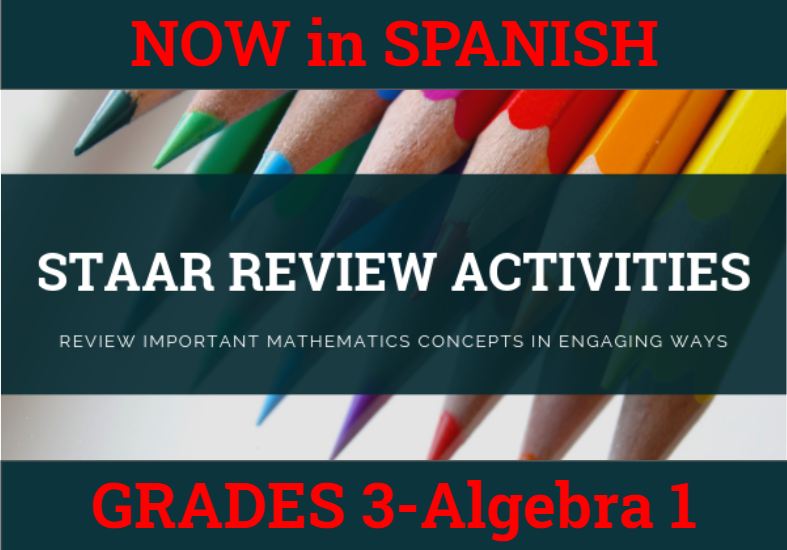How might we purposefully and effectively review important mathematics concepts for the Algebra I EOC STAAR assessment? The ESC-18 Math Team has created a variety of activities where students practice and apply important grade-level TEKS aligned topics to cement their learning.

### Please See Our Pricing and Instructions to Purchase our STAAR Activities

 A.2A A.6A Domain & Range Dominoes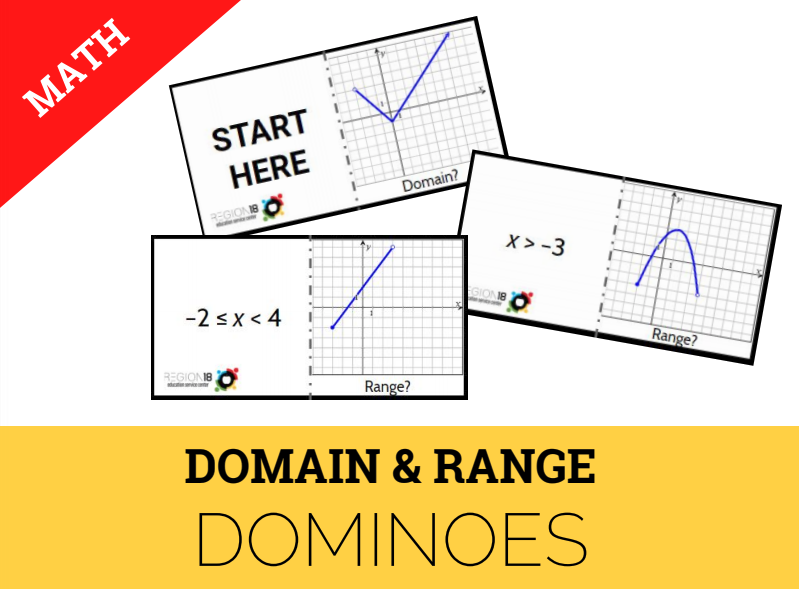Determine the domain and range of linear, quadratic, and exponential functions in mathematical problems and represent the domain and range using inequalities. A.2C Writing Linear Equations Write linear equations in two variables given a table of values, a graph, and a verbal description. A.2I A.5C Writing and Solving Systems of Linear Equations Task Cards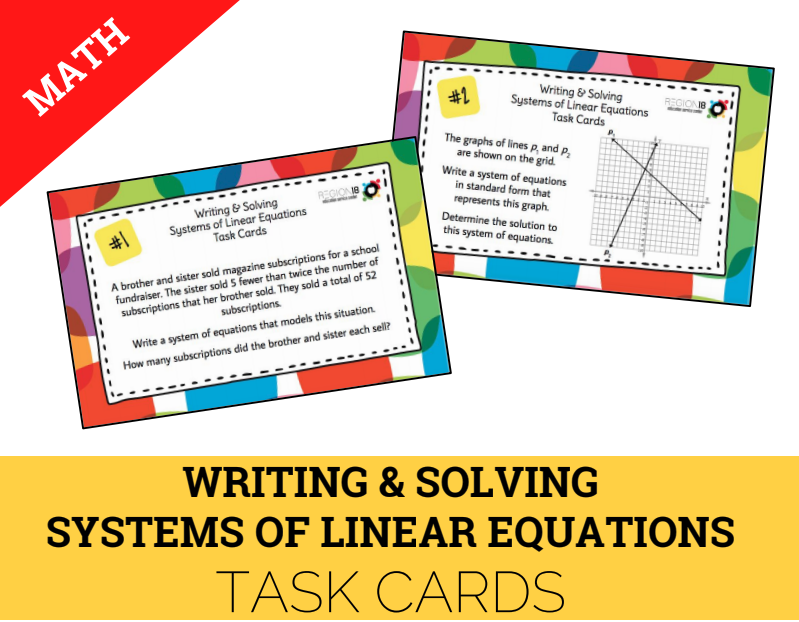Write and solve systems of two linear equations given a table of values, a graph, and a verbal description for mathematical and real-world problems. A.3B Rate of Change Question Stack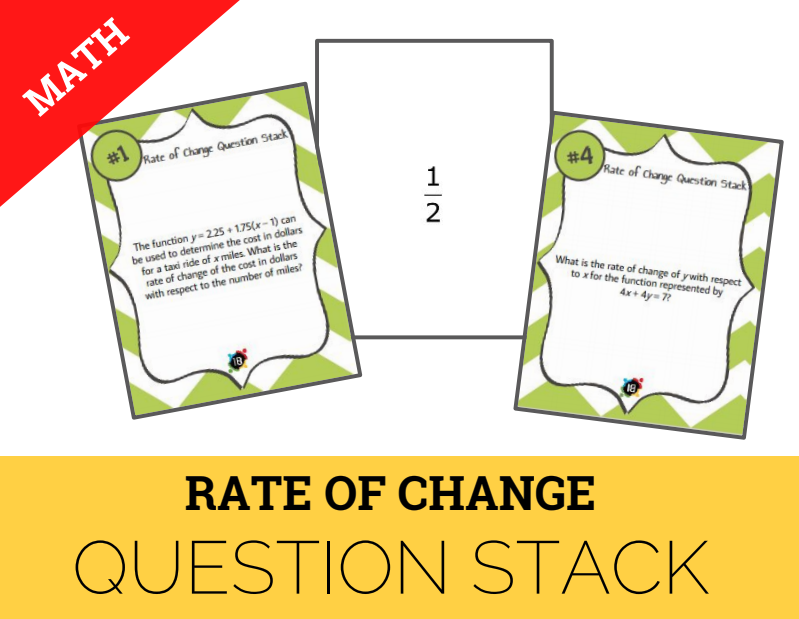Calculate the rate of change of a linear function represented tabularly, graphically, or algebraically in context of mathematical and real-world problems. A.3C A.7A A.9D Key Features of Linear, Quadratic, and Exponential Functions True/False Sort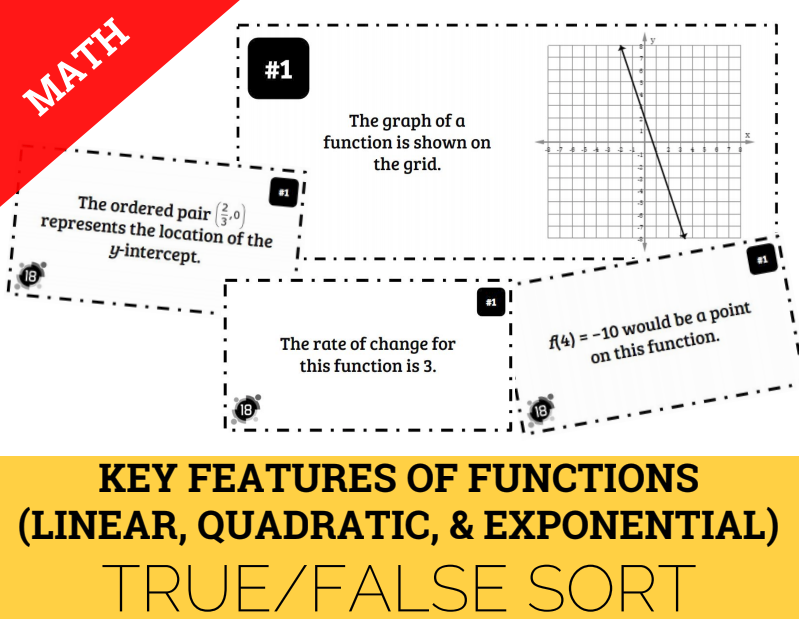Graph linear, quadratic, and exponential functions on the coordinate plane and identify key features in mathematical and real-world problems. A.3D Graphing Linear Inequalities Card Sort Graph the solution set of linear inequalities in two variables on the coordinate plane. A.5A Solving Linear Equations Sum It Up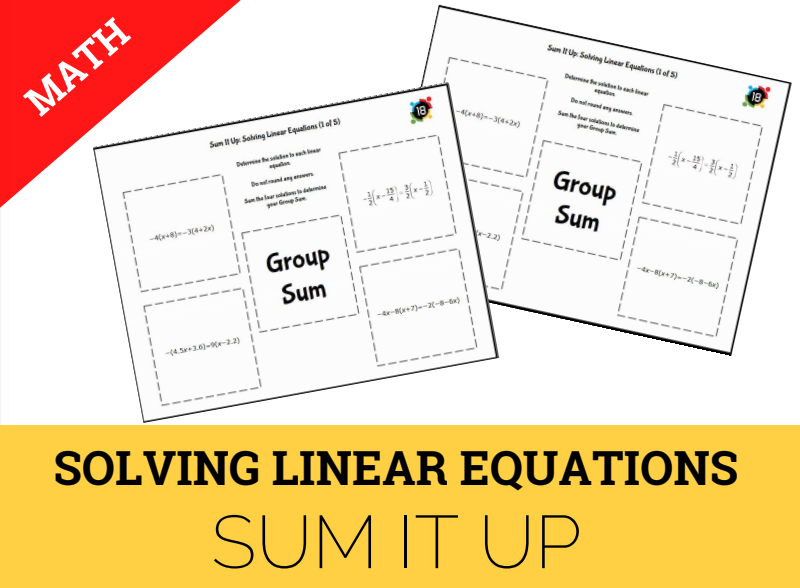Solve linear equations in one variable, including those for which the application of the distributive property is necessary and for which variables are included on both sides. A.8A Solving Quadratic Equations Coloring Page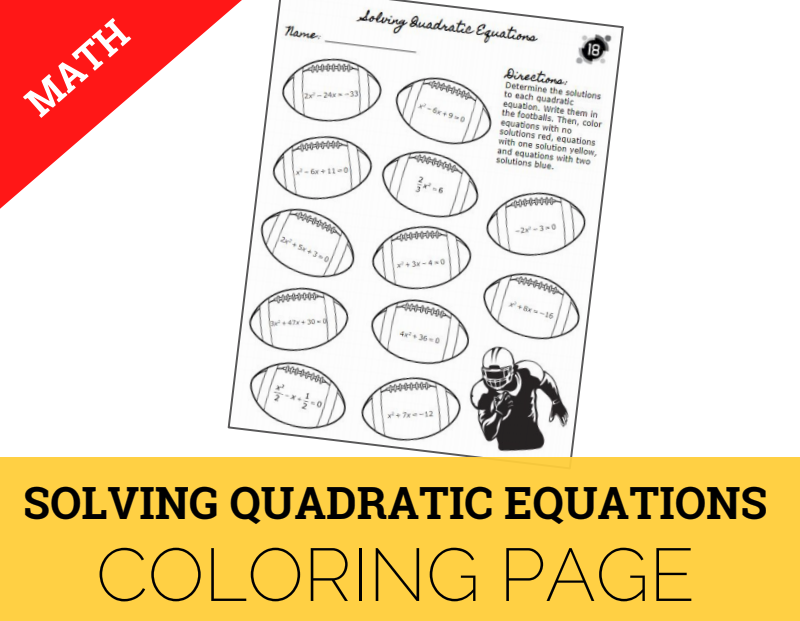Solve quadratic equations having real solutions by factoring, taking square roots, completing the square, and applying the quadratic formula. A.9C, A.12C, A.12D Writing Exponential Functions Quick Flips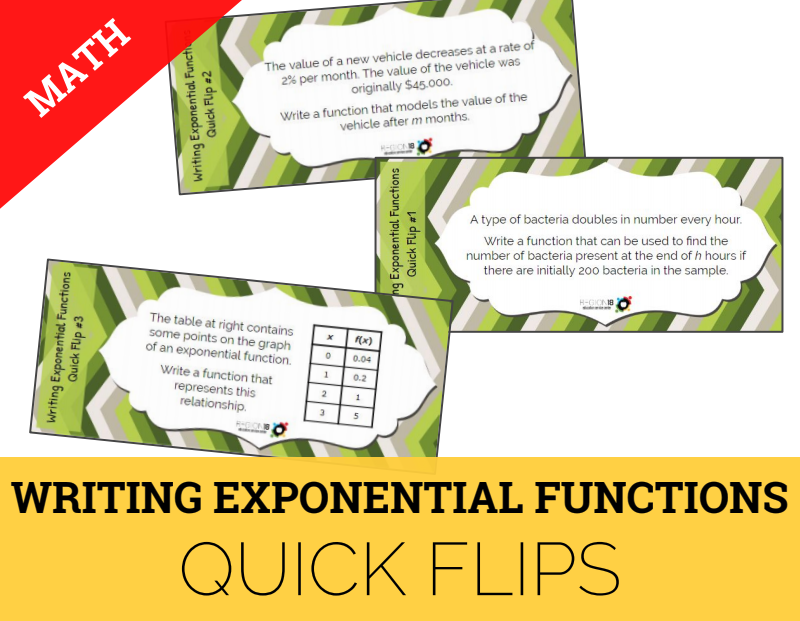Write exponential functions in the form f(x) = abx to describe problems arising from mathematical and real-world situations, including growth and decay. A.10E A.10F Factoring Quadratic Expressions Question Stack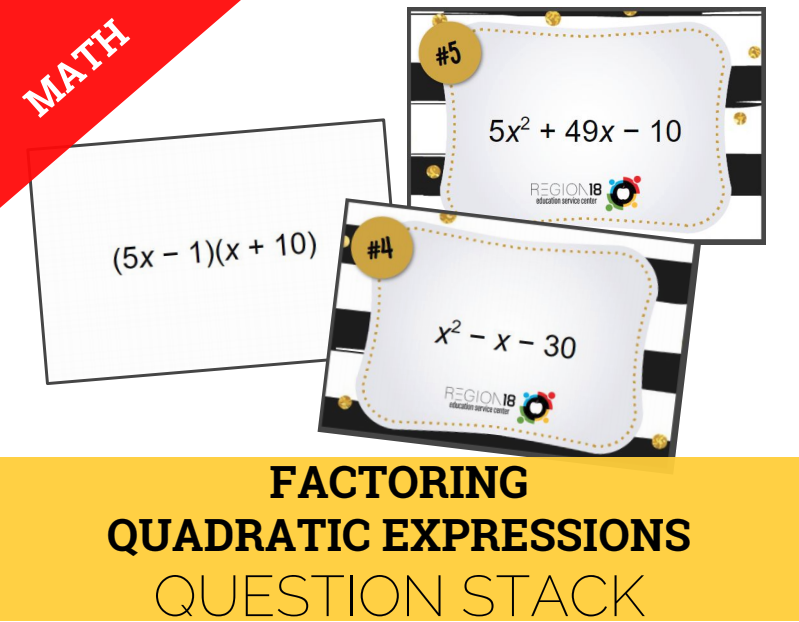Factor trinomials with real factors in the form ax2 + bx + c, including perfect square trinomials of degree two. Factor binomials that represent a difference of squares.